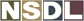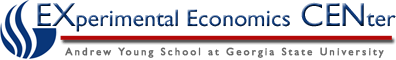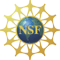Students / Subjects# Examples of Calculating GDP

Here, we will show you the two different ways of calculating GDP using the information from different factors given in Table 1.

### Using the Expenditures Approach

#### Table 1: Expenditures

 Transfer Payments \$54 Interest Income \$150 Depreciation \$36 Wages \$67 Gross Private Investment (I) \$124 Business Profits \$200 Indirect Business Taxes \$74 Rental Income \$75 Net Exports (X-M) \$18 Net Foreign Factor Income \$12 Government Purchases (G) \$156 Household Consumption (C) \$304

By using the data in Table 1 we can calculate the GDP using the expenditures approach. As you can see, the table contains more data than is necessary so you have to look for the parts which make up the expenditures approach to calculating GDP.  The necessary data is highlighted within the table.
Remember:

GDP = C + G + I + (X - M)

In this case the C is represented by Household Consumption which is \$304.
The G refers to Government Spending which is \$156.
I is gross private investment and is \$124.
(X - M) is the net exports and in the table is shown to be \$18.

Therefore:

GDP = \$304 + \$156 + \$124 + \$18

GDP = \$602

### Using the Income Approach

Table 1 also contains the data necessary to calculate GDP using the income approach.

Table 1: Income
 Transfer Payments \$54 Interest Income (i) \$150 Depreciation \$36 Wages (W) \$67 Gross Private Investment \$124 Business Profits (PR) \$200 Indirect Business Taxes \$74 Rental Income (R) \$75 Net Exports \$18 Net Foreign Factor Income \$12 Government Purchases \$156 Household Consumption \$304

In this case we use the formula:

NI = W + R + i + PR

W is the wages that are represented by \$67 in the table.
Rental income is the R and is \$75.
Interest income is i and is \$150.
PR are

Therefore:

NI = \$67 + \$75 + \$150 + \$200

NI = \$492

GDP = NI + Indirect Business Taxes + Depreciation

GDP = \$492 + \$74 + \$36

GDP = \$602

As you can see, in this case, both approaches to calculating GDP will give the same estimate. This is not always what happens and sometimes GDP will differ slightly when the different approaches are used.